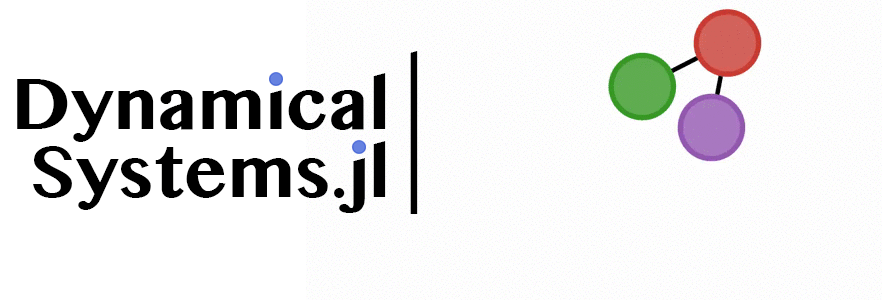Award-winning feature-full library for the exploration of dynamical systems and chaos.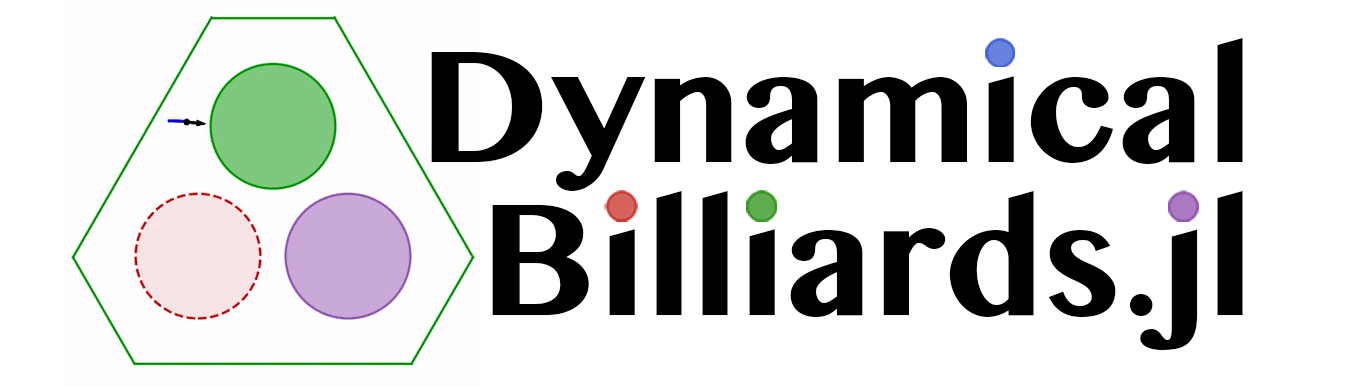An easy-to-use, modular, performant and extendable package for dynamical billiards in two dimensions.Software that helps scientists deal with their scientific projects.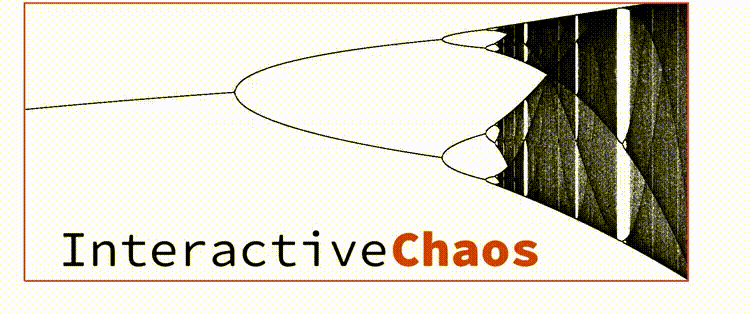Interactive applications for the exploration of chaos and dynamical systems.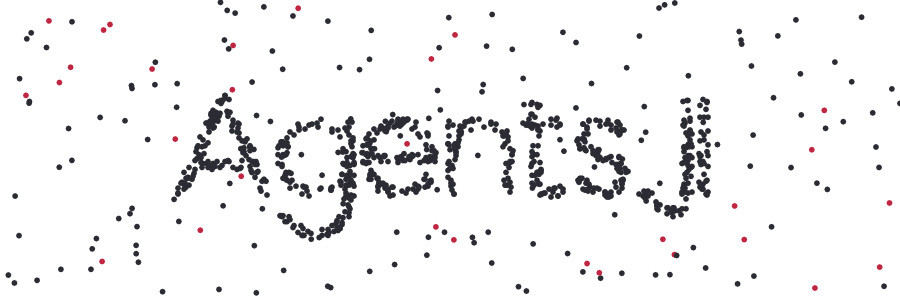Agent-based modelling framework, including visualization and data collection functionality.Prediction of timeseries using methods of nonlinear dynamics and timeseries analysis.

### Simple

All packages are intuitive, simple to use and simple to understand. We also take extra care to write simple and concise source code.

### Well Documented

Every package is accompanied with its own dedicated documentation which is automatically generated, always up-to-date and full of examples. In addition all exported functions have detailed documentation strings. Click the logo of each package to access the documentation.

### Open Source

All packages are open source and hosted on GitHub! Our packages are also licenced under very permissive licenses (MIT or GPL-3.0). Want to contribute? Check out the GitHub repos of the individual packages!

using DynamicalBilliards, PyPlot

N = 20
cs = [(i/N, 0, 1 - i/N, 0.5) for i in 1:N]
ps = [Particle(1, 0.6 + 0.0005*i, 0) for i in 1:N]

animate_evolution(ps, bd, 7.0; colors = cs, tailtime = 1.5)

using DynamicalBilliards, PyPlot

# Create a circular "lens"
o = Antidot(SVector(1.0, 0.75), 0.5)
bd = Billiard(billiard_rectangle(2.5, 1.5)..., o)

trans, refra = law_of_refraction(1.5)
rs = (RaySplitter(, trans, refra),)

ps = [Particle(0.1, y, 0.0) for y in 0.4:0.05:1.1]; N = length(ps)
cs = [0.5 .* (0, i/N, 1 - i/N, 0.9) for i in eachindex(ps)]

animate_evolution(ps, bd, 2.0, rs, tailtime=2.5, colors = cs)

using DynamicalBilliards, PyPlot

bd = billiard_hexagonal_sinai(0.4, 1.0; setting = "periodic")

p = MagneticParticle(0.5, 0.6, π/2, 0.75)

xt, yt = timeseries(p, bd, 15)

plot(bd, xt, yt; hexagonal = true)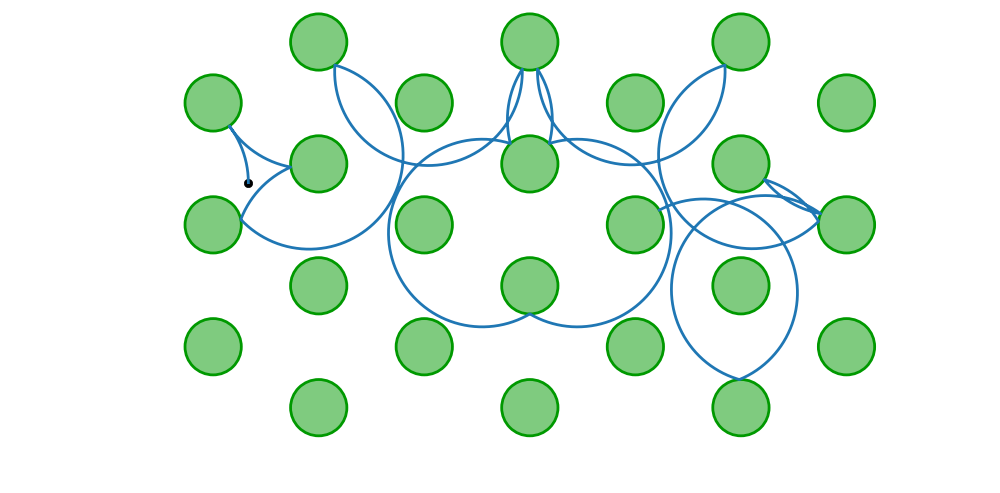using DynamicalSystems, PyPlot

function roessler(u, p, t)
a, b, c = p
du1 = -u-u
du2 = u + a*u
du3 = b + u*(u - c)
return SVector{3}(du1, du2, du3)
end
ds = ContinuousDynamicalSystem(roessler, [0, 1.0, 0], [0.2, 0.2, 5.7])

cs = 4:0.01:6; λs = zeros(length(cs), 3)
for (i, c) in enumerate(cs)
set_parameter!(ds, 3, c)
λs[i, :] .= lyapunovs(ds, 10000; Ttr = 500.0)
end

plot(cs, λs)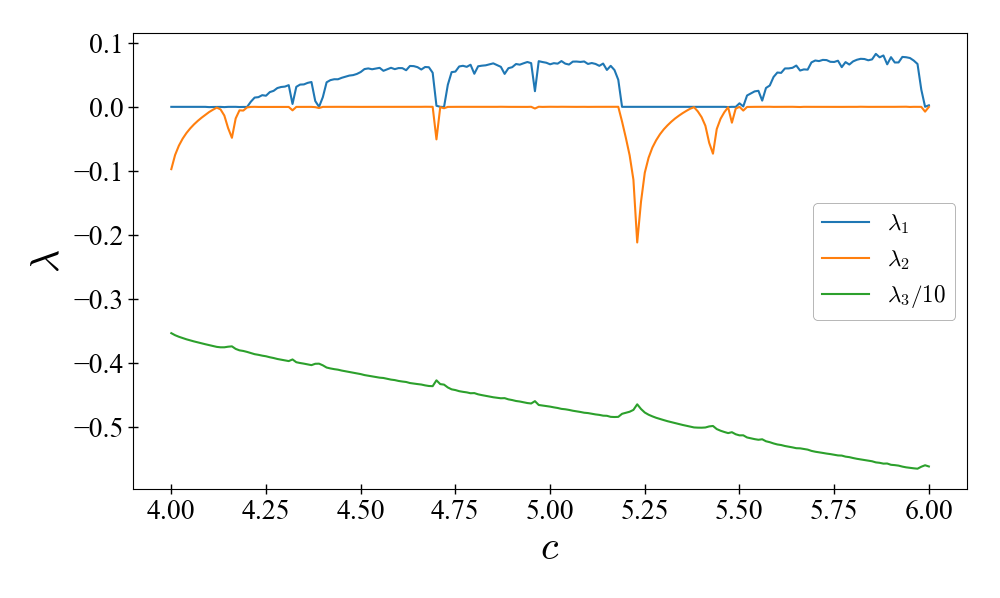using DynamicalSystems, PyPlot

ds = Systems.roessler()
tr = trajectory(ds, 1000.0; dt = 0.05)

τ = estimate_delay(tr[:, 1], "mi_min") # first minimum of mutual information

figure(figsize = (10, 6)); subplot(1,2,1)
for method in ["afnn", "fnn", "f1nn"]
Ds = estimate_dimension(tr[:, 1], τ, 1:6, method)
plot(1:6, Ds ./ maximum(Ds), label = method, marker = "o")
end
legend(); xlabel("\$\\gamma\$ (temporal neighbors)")

subplot(1,2,2, projection = "3d")
rec = embed(tr[:, 1], 4, τ)
plot3D(rec[1:2000, 1],rec[1:2000, 2],rec[1:2000, 3])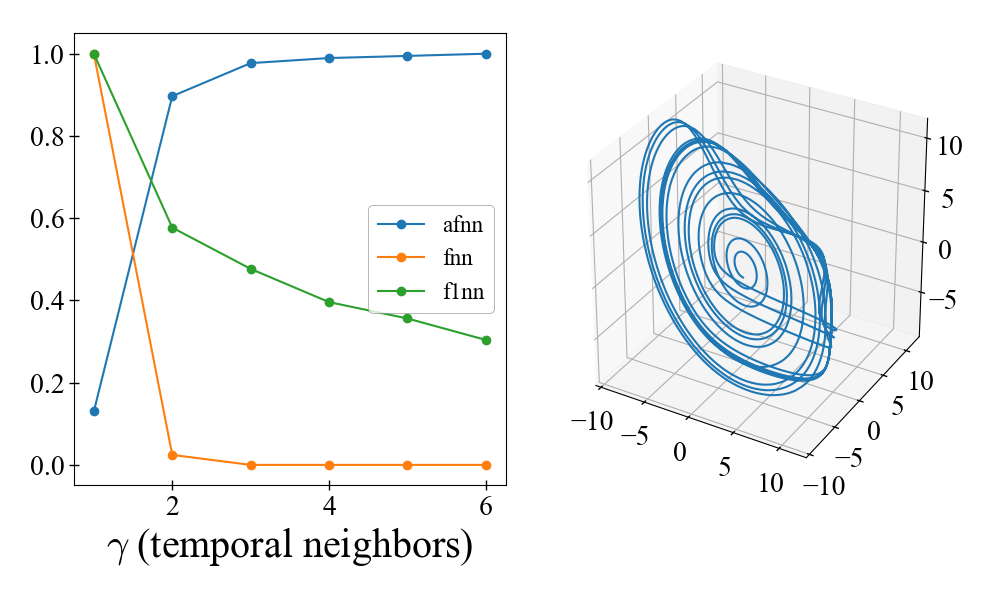using DynamicalSystems, PyPlot

ds = Systems.roessler()

for (i, c) in enumerate([4.0, 5.7])
set_parameter!(ds, 3, c)
tr = trajectory(ds, 200.0; dt = 0.1, Ttr = 200.0)

R = RecurrenceMatrix(tr, 3.0)

subplot(1,2,i)
imshow(grayscale(R; width = 1000, height = 1000), cmap = "binary_r")
end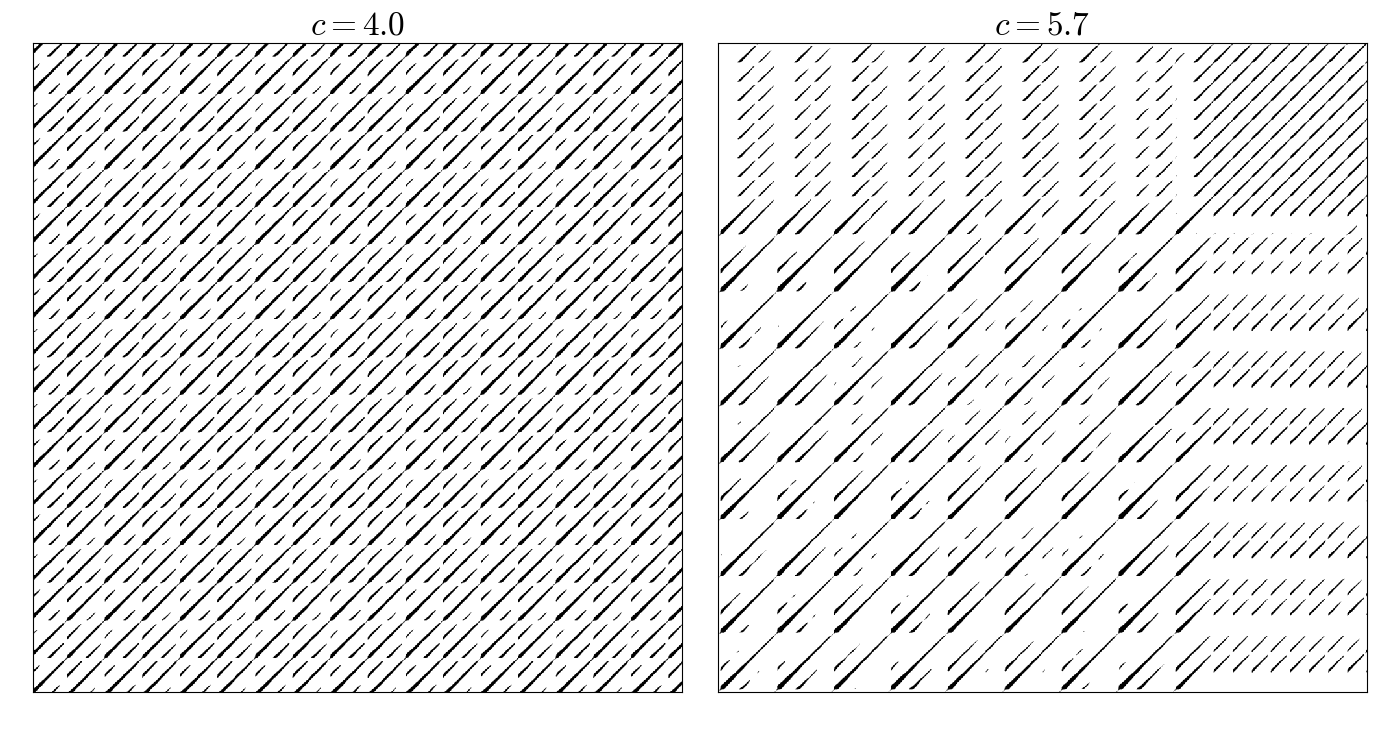using DynamicalSystems, PyPlot, OrdinaryDiffEq

hh = Systems.henonheiles()

plane = (1, 0.0)
u0s = [[0.0, -0.25, 0.42081, 0.0],
[0.0, -0.31596, 0.354461, 0.0591255],
[0.0, 0.1, 0.5, 0.0],
[0.0, -0.0910355, 0.459522, -0.173339],
[0.0, -0.205144, 0.449328, -0.0162098]]

for u0 in u0s
psos = poincaresos(hh, plane, 20000.0; alg = Vern9(), u0 = u0)
scatter(psos[:, 2], psos[:, 4], s = 2.0)
end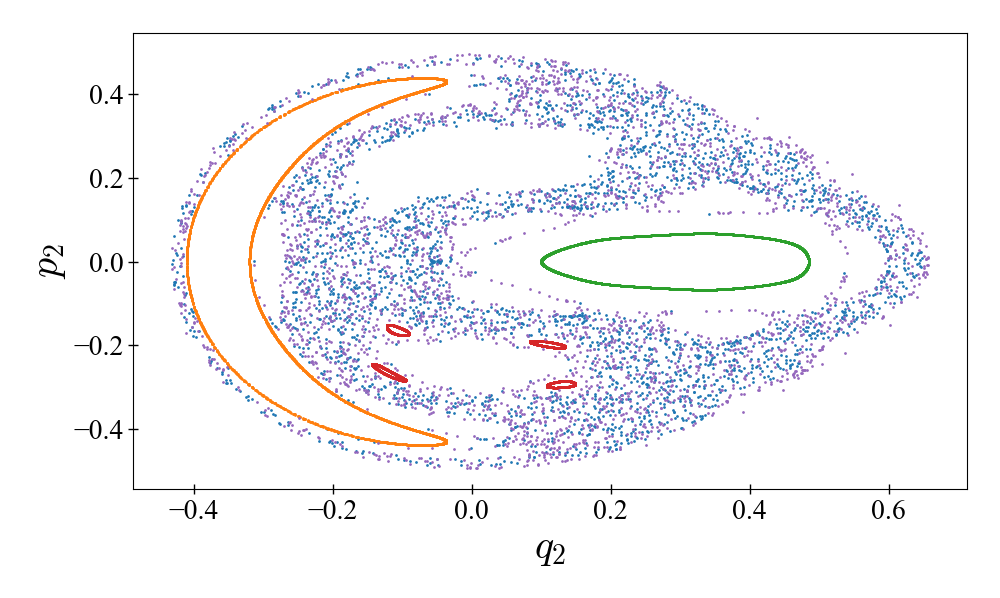using InteractiveChaos, Makie, DynamicalSystems

i = 1
p_index = 1

ds, p_min, p_max, parname = Systems.henon(), 0.8, 1.4, "a"
t = "orbit diagram for the Hénon map"

interactive_orbitdiagram(ds, p_index, p_min, p_max;
parname = parname, title = t)

using Agents, AgentsPlots
pyplot()

model, agent_step!, model_step! = Models.flocking()

function bird_shape(b)
φ = atan(b.vel, b.vel)
xs = [(i ∈ (0, 3) ? 2 : 1) * cos(i * 2π / 3 + φ) for i in 0:3]
ys = [(i ∈ (0, 3) ? 2 : 1) * sin(i * 2π / 3 + φ) for i in 0:3]
Shape(xs, ys)
end
bird_color(a) = RGB(0.5, 0, mod1(a.id, 20)/20)

anim = @animate for i in 1:200
p1 = plotabm(model;
ac = bird_color, am = bird_shape, as = 10, msw = 0,
xlims = (0, 100), ylims = (0, 100))
step!(model, agent_step!, model_step!, 1)
end
mp4(anim, "flocking.mp4", fps = 25)


All packages of JuliaDynamics are registered! To install them simply press ] to enter the package manager mode and then do: add PackageName. To get started with Julia, check out Julia's setup instructions. For plotting we recommend PyPlot or Makie.